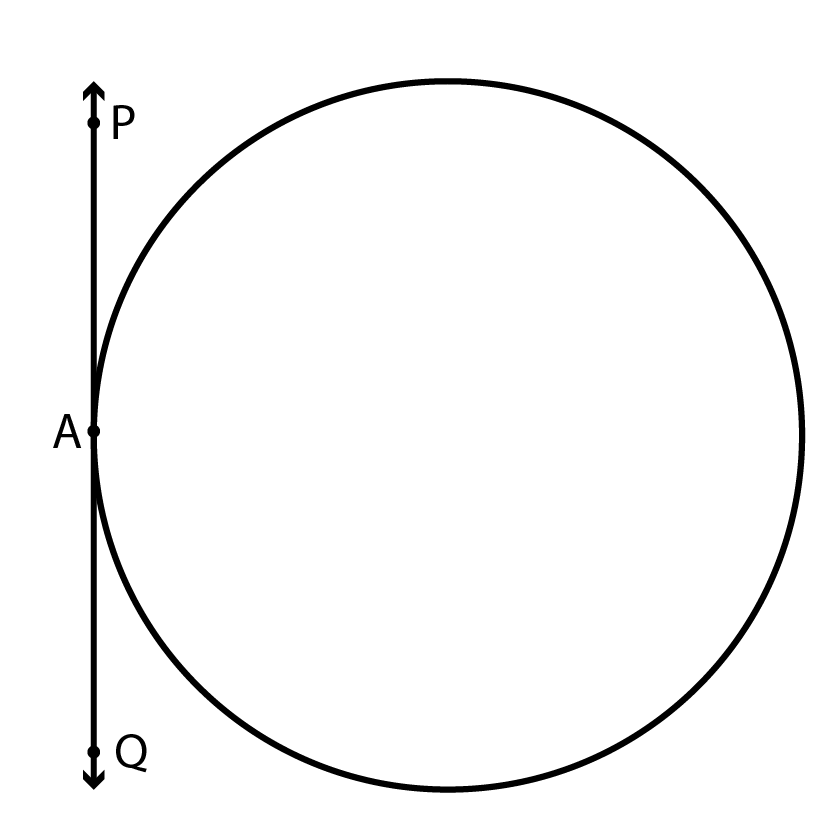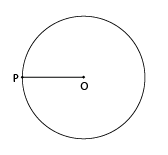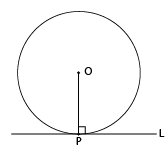## Objective

To verify that the tangent at any point to a circle is perpendicular to the radius through that point of contact.

## Theory

### 1) What is tangent?

A line that touches the circle at a single point is known as tangent to a circle.Fig. (A) Tangent to the circle

### 2) What is the radius of a circle?

The distance from the centre of a circle to the outside edge. See Fig (B).Fig. (B) Radius of the circle

### 3) What is perpendicular angle?

Perpendicular lines are lines that intersect at a right (90˚) angle. See Fig (C).Fig (C) Perpendicular angle

### 4) Maths rule:

Shortest distance between a point and line is perpendicular distance.